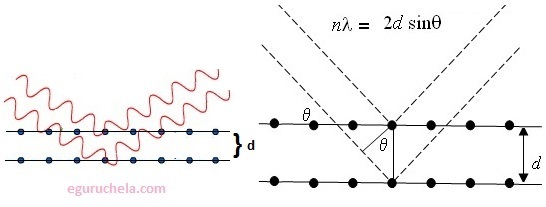# Bragg’s equation

Bragg’s Law was introduced by Sir W.H. Bragg and his son Sir W.L. Bragg. The structures of crystals and molecules are often being identified using x-ray diffraction studies, which are explained by Bragg’s Law. The law states that when the x-ray is incident onto a crystal surface, its angle of incidence, θ, will reflect back with a same angle of scattering, θ. And, when the path difference, d is equal to a whole number, n, of wavelength, a constructive interference will occur.

The Bragg's Law equation : nΛ = 2d sinΘ

where,

Λ = the wavelength of the x-ray

d = the spacing of the crystal layers (path difference)

θ = the incident angle (the angle between incident ray and the scatter plane)

n = an integer### Example

• Inter planar distance between two layers is 4.00A in a crystal. Calculate the angle of reflection for first order reflection. X-rays of wavelength 1.50A are diffracted by the crystal.
N lambda = 2d sin theta
(1)(1.540A)=(2)(40A)sin theta
Θ = sin-10.1925
Θ = 11.1theta

Go to index page